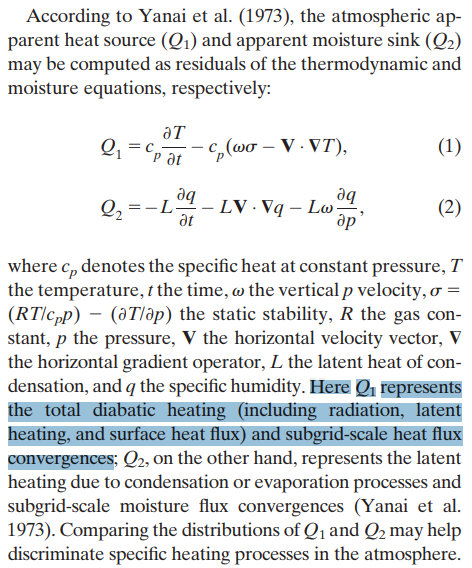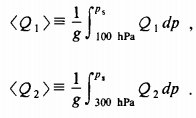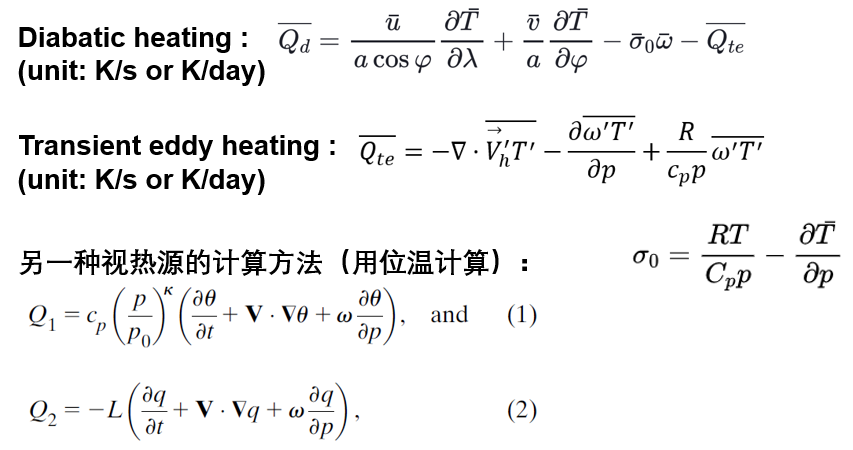RQL's Blog 菜鸟成长记

# 大气视热源

2019-02-01
renql

the vertically integrated atmospheric apparent heat source 整层积分的大气视热源CESM会默认输出月平均的上述三个加热过程的物理量，如下所示。把他们都加起来乘以Cp再整层积分后得到的值与用上述公式（1）得到的结果差不多。也可以分别看每一项对加热的贡献。

``````    float QRL(time, lev, lat, lon) ;
QRL:mdims = 1 ;
QRL:units = "K/s" ;
QRL:long_name = "Longwave heating rate" ;
QRL:cell_methods = "time: mean" ;

float QRS(time, lev, lat, lon) ;
QRS:mdims = 1 ;
QRS:units = "K/s" ;
QRS:long_name = "Solar heating rate" ;
QRS:cell_methods = "time: mean" ;

float DTCOND(time, lev, lat, lon) ;
DTCOND:mdims = 1 ;
DTCOND:units = "K/s" ;
DTCOND:long_name = "T tendency - moist processes" ;
DTCOND:cell_methods = "time: mean" ;

float DTV(time, lev, lat, lon) ;
DTV:mdims = 1 ;
DTV:units = "K/s" ;
DTV:long_name = "T vertical diffusion" ;
DTV:cell_methods = "time: mean" ;
``````

Content